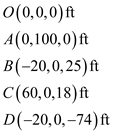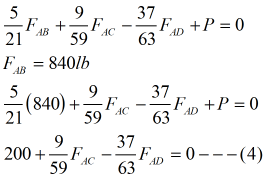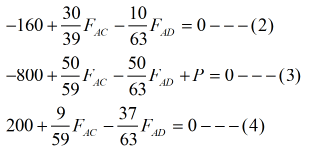# A transmission tower is held by three guy wires attached to a pin at A and anchored by bolts at B, C, and D. If the tension in wire AB is 840lb, determine the vertical force P exerted by the tower on the pin at A.

Question-AnswerCategory: Engineering MechanicsA transmission tower is held by three guy wires attached to a pin at A and anchored by bolts at B, C, and D. If the tension in wire AB is 840lb, determine the vertical force P exerted by the tower on the pin at A.

### A transmission tower is held by three guy wires attached to a pin at A and anchored by bolts at B, C, and D. If the tension in wire AB is 840lb, determine the vertical force P exerted by the tower on the pin at A.Step 1
Write the coordinates of different points of the system.Calculation of position vector AB and tension in ABStep 3

Calculation of position vector AC and tension in ACStep 4Step 5

Apply equilibrium of forcesEquate the component of i in equation (1)Equate the component of j in equation (1)Equate the component of k in equation (1)Step 6

Solving equation (2) , (3) and (4)The vertical force exerted by the tower is P = 1553lb